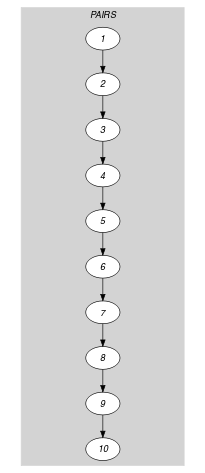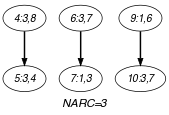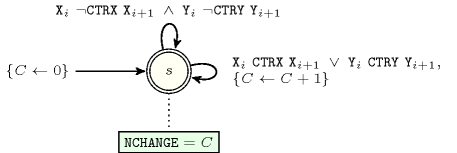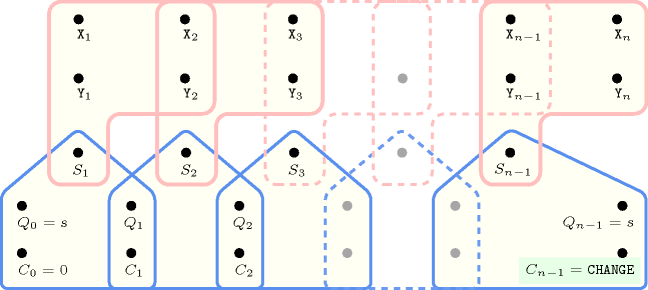## 5.63. change_pair

Origin

Derived from $\mathrm{𝚌𝚑𝚊𝚗𝚐𝚎}$.

Constraint

$\mathrm{𝚌𝚑𝚊𝚗𝚐𝚎}_\mathrm{𝚙𝚊𝚒𝚛}\left(\mathrm{𝙽𝙲𝙷𝙰𝙽𝙶𝙴},\mathrm{𝙿𝙰𝙸𝚁𝚂},\mathrm{𝙲𝚃𝚁𝚇},\mathrm{𝙲𝚃𝚁𝚈}\right)$

Arguments
 $\mathrm{𝙽𝙲𝙷𝙰𝙽𝙶𝙴}$ $\mathrm{𝚍𝚟𝚊𝚛}$ $\mathrm{𝙿𝙰𝙸𝚁𝚂}$ $\mathrm{𝚌𝚘𝚕𝚕𝚎𝚌𝚝𝚒𝚘𝚗}\left(𝚡-\mathrm{𝚍𝚟𝚊𝚛},𝚢-\mathrm{𝚍𝚟𝚊𝚛}\right)$ $\mathrm{𝙲𝚃𝚁𝚇}$ $\mathrm{𝚊𝚝𝚘𝚖}$ $\mathrm{𝙲𝚃𝚁𝚈}$ $\mathrm{𝚊𝚝𝚘𝚖}$
Restrictions
 $\mathrm{𝙽𝙲𝙷𝙰𝙽𝙶𝙴}\ge 0$ $\mathrm{𝙽𝙲𝙷𝙰𝙽𝙶𝙴}<|\mathrm{𝙿𝙰𝙸𝚁𝚂}|$ $\mathrm{𝚛𝚎𝚚𝚞𝚒𝚛𝚎𝚍}$$\left(\mathrm{𝙿𝙰𝙸𝚁𝚂},\left[𝚡,𝚢\right]\right)$ $\mathrm{𝙲𝚃𝚁𝚇}\in \left[=,\ne ,<,\ge ,>,\le \right]$ $\mathrm{𝙲𝚃𝚁𝚈}\in \left[=,\ne ,<,\ge ,>,\le \right]$
Purpose

$\mathrm{𝙽𝙲𝙷𝙰𝙽𝙶𝙴}$ is the number of times that the following disjunction holds: $\left({X}_{1}\mathrm{𝙲𝚃𝚁𝚇}{X}_{2}\right)\vee \left({Y}_{1}\mathrm{𝙲𝚃𝚁𝚈}{Y}_{2}\right)$, where $\left({X}_{1},{Y}_{1}\right)$ and $\left({X}_{2},{Y}_{2}\right)$ correspond to consecutive pairs of variables of the collection $\mathrm{𝙿𝙰𝙸𝚁𝚂}$.

Example
$\left(\begin{array}{c}3,〈\begin{array}{cc}𝚡-3\hfill & 𝚢-5,\hfill \\ 𝚡-3\hfill & 𝚢-7,\hfill \\ 𝚡-3\hfill & 𝚢-7,\hfill \\ 𝚡-3\hfill & 𝚢-8,\hfill \\ 𝚡-3\hfill & 𝚢-4,\hfill \\ 𝚡-3\hfill & 𝚢-7,\hfill \\ 𝚡-1\hfill & 𝚢-3,\hfill \\ 𝚡-1\hfill & 𝚢-6,\hfill \\ 𝚡-1\hfill & 𝚢-6,\hfill \\ 𝚡-3\hfill & 𝚢-7\hfill \end{array}〉,\ne ,>\hfill \end{array}\right)$

In the example we have the following 3 changes:

• One change between pairs $𝚡-3$ $𝚢-8$ and $𝚡-3$ $𝚢-4$ since $3\ne 3\vee 8>4$,

• One change between pairs $𝚡-3$ $𝚢-7$ and $𝚡-1$ $𝚢-3$ since $3\ne 1\vee 7>3$,

• One change between pairs $𝚡-1$ $𝚢-6$ and $𝚡-3$ $𝚢-7$ since $1\ne 3\vee 6>7$.

Consequently the $\mathrm{𝚌𝚑𝚊𝚗𝚐𝚎}_\mathrm{𝚙𝚊𝚒𝚛}$ constraint holds since its first argument $\mathrm{𝙽𝙲𝙷𝙰𝙽𝙶𝙴}$ is assigned value 3.

Typical
 $\mathrm{𝙽𝙲𝙷𝙰𝙽𝙶𝙴}>0$ $|\mathrm{𝙿𝙰𝙸𝚁𝚂}|>1$ $\mathrm{𝚛𝚊𝚗𝚐𝚎}$$\left(\mathrm{𝙿𝙰𝙸𝚁𝚂}.𝚡\right)>1$ $\mathrm{𝚛𝚊𝚗𝚐𝚎}$$\left(\mathrm{𝙿𝙰𝙸𝚁𝚂}.𝚢\right)>1$
Symmetries
• One and the same constant can be added to the $𝚡$ attribute of all items of $\mathrm{𝙿𝙰𝙸𝚁𝚂}$.

• One and the same constant can be added to the $𝚢$ attribute of all items of $\mathrm{𝙿𝙰𝙸𝚁𝚂}$.

Arg. properties

Functional dependency: $\mathrm{𝙽𝙲𝙷𝙰𝙽𝙶𝙴}$ determined by $\mathrm{𝙿𝙰𝙸𝚁𝚂}$, $\mathrm{𝙲𝚃𝚁𝚇}$ and $\mathrm{𝙲𝚃𝚁𝚈}$.

Usage

Here is a typical example where this constraint is useful. Assume we have to produce a set of cables. A given quality and a given cross-section that respectively correspond to the $𝚡$ and $𝚢$ attributes of the previous pairs of variables characterise each cable. The problem is to sequence the different cables in order to minimise the number of times two consecutive wire cables ${C}_{1}$ and ${C}_{2}$ verify the following property: ${C}_{1}$ and ${C}_{2}$ do not have the same quality or the cross section of ${C}_{1}$ is greater than the cross section of ${C}_{2}$.

generalisation: $\mathrm{𝚌𝚑𝚊𝚗𝚐𝚎}_\mathrm{𝚟𝚎𝚌𝚝𝚘𝚛𝚜}$ ($\mathrm{𝚙𝚊𝚒𝚛}$ of $\mathrm{𝚟𝚊𝚛𝚒𝚊𝚋𝚕𝚎𝚜}$ replaced by $\mathrm{𝚟𝚎𝚌𝚝𝚘𝚛}$).

specialisation: $\mathrm{𝚌𝚑𝚊𝚗𝚐𝚎}$ ($\mathrm{𝚙𝚊𝚒𝚛}$ of $\mathrm{𝚟𝚊𝚛𝚒𝚊𝚋𝚕𝚎𝚜}$ replaced by $\mathrm{𝚟𝚊𝚛𝚒𝚊𝚋𝚕𝚎}$).

Keywords
Arc input(s)

$\mathrm{𝙿𝙰𝙸𝚁𝚂}$

Arc generator
$\mathrm{𝑃𝐴𝑇𝐻}$$↦\mathrm{𝚌𝚘𝚕𝚕𝚎𝚌𝚝𝚒𝚘𝚗}\left(\mathrm{𝚙𝚊𝚒𝚛𝚜}\mathtt{1},\mathrm{𝚙𝚊𝚒𝚛𝚜}\mathtt{2}\right)$

Arc arity
Arc constraint(s)
$\mathrm{𝚙𝚊𝚒𝚛𝚜}\mathtt{1}.𝚡\mathrm{𝙲𝚃𝚁𝚇}\mathrm{𝚙𝚊𝚒𝚛𝚜}\mathtt{2}.𝚡\vee \mathrm{𝚙𝚊𝚒𝚛𝚜}\mathtt{1}.𝚢\mathrm{𝙲𝚃𝚁𝚈}\mathrm{𝚙𝚊𝚒𝚛𝚜}\mathtt{2}.𝚢$
Graph property(ies)
$\mathrm{𝐍𝐀𝐑𝐂}$$=\mathrm{𝙽𝙲𝙷𝙰𝙽𝙶𝙴}$

Graph class
 $•$$\mathrm{𝙰𝙲𝚈𝙲𝙻𝙸𝙲}$ $•$$\mathrm{𝙱𝙸𝙿𝙰𝚁𝚃𝙸𝚃𝙴}$ $•$$\mathrm{𝙽𝙾}_\mathrm{𝙻𝙾𝙾𝙿}$

Graph model

Same as $\mathrm{𝚌𝚑𝚊𝚗𝚐𝚎}$, except that each item has two attributes $𝚡$ and $𝚢$.

Parts (A) and (B) of Figure 5.63.1 respectively show the initial and final graph associated with the Example slot. Since we use the $\mathrm{𝐍𝐀𝐑𝐂}$ graph property, the arcs of the final graph are stressed in bold.

##### Figure 5.63.1. Initial and final graph of the $\mathrm{𝚌𝚑𝚊𝚗𝚐𝚎}_\mathrm{𝚙𝚊𝚒𝚛}$ constraint(a) (b)
Automaton

Figure 5.63.2 depicts the automaton associated with the $\mathrm{𝚌𝚑𝚊𝚗𝚐𝚎}_\mathrm{𝚙𝚊𝚒𝚛}$ constraint. To each pair of consecutive pairs $\left(\left({𝚇}_{i},{𝚈}_{i}\right),\left({𝚇}_{i+1},{𝚈}_{i+1}\right)\right)$ of the collection $\mathrm{𝙿𝙰𝙸𝚁𝚂}$ corresponds a 0-1 signature variable ${S}_{i}$. The following signature constraint links ${𝚇}_{i}$, ${𝚈}_{i}$, ${𝚇}_{i+1}$, ${𝚈}_{i+1}$ and ${S}_{i}$: $\left({𝚇}_{i}\mathrm{𝙲𝚃𝚁𝚇}{𝚇}_{i+1}\right)\vee \left({𝚈}_{i}\mathrm{𝙲𝚃𝚁𝚈}{𝚈}_{i+1}\right)⇔{S}_{i}$.

##### Figure 5.63.2. Automaton of the $\mathrm{𝚌𝚑𝚊𝚗𝚐𝚎}_\mathrm{𝚙𝚊𝚒𝚛}$ constraint##### Figure 5.63.3. Hypergraph of the reformulation corresponding to the automaton of the $\mathrm{𝚌𝚑𝚊𝚗𝚐𝚎}_\mathrm{𝚙𝚊𝚒𝚛}$ constraint